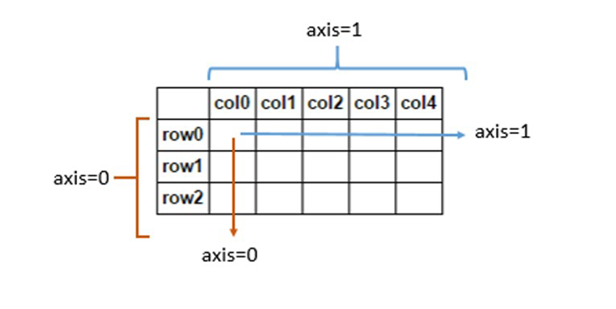signed

QiShunwang

“诚信为本、客户至上”

# Tensorflow 函数 API 中 axis轴的理解

2021/5/15 1:04:39   来源：

Tensorflow 就是对 tensor进行一系列的操作，今天来讲解在很多tensorflow API中都存在的 axis 参数。

tensorflow 中的 axis 和 numpy 中的 axis 是一样的axis是跟shape相关的。

axis = 0就是指a这个维度，
axis = 2就是指c这个维度，
axis = -1就是指d这个维度。（-1在python里指最后一个）

tf.argmax(x, axis=0)表示batch维的方向
tf.argmax(x, axis=1)表示height维的方向
tf.argmax(x, axis=2)表示width维的方向
tf.argmax(x, axis=3)表示channels维的方向
tf.argmax(x, axis=-1)同表示channels维的方向

numpy中的axis同Tensorflow中的axis意义一样。